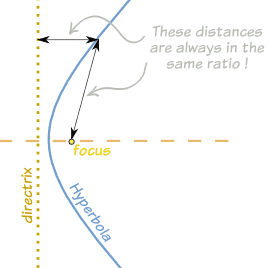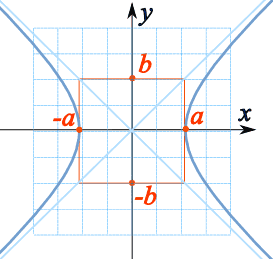# What is meant by the eccentricity of a hyperbola?

Aug 30, 2015

The eccentricity of a hyperbola is the ratio of the distance from any point on the graph to (a) the focus and (b) the directrix.

#### Explanation:

A hyperbola is a curve where the distances of any point from a fixed point (the focus) and a fixed straight line (the directrix) are always in the same ratio.This ratio is called the eccentricity $e$.

The equation for a hyperbola is:

x^2/a^2 − y^2/b^2 = 1The formula for eccentricity $e$ is

$e = \frac{\sqrt{{a}^{2} + {b}^{2}}}{a}$

The eccentricity measures the degree of opening of the branches of the hyperbola.

The greater the eccentricity, the more open the arms of the hyperbola.